Our World Statistics Day conversations have been a great reminder of how much statistics can inform our lives. Do you have an example of how statistics has made a difference in your life? Share your story with the Community!
Choose Language Hide Translation Bar
What Good Are Error Bars?

A cognitive psychology blog recently published an interesting post about an important and long-standing problem with scientific publications: Most researchers don't understand error bars.

Error bars are graphical elements included in a statistical plot to represent the uncertainty in a sample statistic. They are important to JMP users, as error bars are commonly mentioned when users are asked about what new features they want to see in the next version of the software.

They have been used for a long time, yet there is no real standard for them. Many publications have adopted some conventions over time, but no harmonization has occurred to date. The name "error bar" might imply that it represents one standard error, but in practice, error bars take various forms and represent other quantities, such as the sample standard deviation or a confidence interval of the sample mean. Different "rules of thumb" abound about how to make or interpret them. Whether you are an author selecting error bars for your plots or a reader interpreting error bars in an article, you might experience some confusion about their purpose and form.

The author of the blog, Dave Munger, polled readers before writing his entry to see if they knew how to use these statistical graphics and was not surprised to find that many participants had difficulty. He cited a large published study by Sarah Belia that found the same outcome for authors in psychology, neuroscience, and medical journals. How could intelligent, highly trained, and experienced researchers have difficulty with such an old, common, and seemingly simple device?

I think that the reason for this common professional malady is that we have used the same device for different purposes, we have used different graphics to represent the same thing, and we have used different formulae to compute them. To see this, compare error bars to another graphic device that is also used to represent uncertainty in sample statistics, the Shewhart control chart. This family of charts has an explicit model for each kind of sample statistic (e.g., individual data, sample average, sample range).

Control charts have rigorous rules for computing control limits and making the chart. Every chart is made of the same components so that if you learn how to read a sample means chart (Xbar chart), for example, you can also read a proportion defective chart (P chart). While the chart type depends on the data, the chart is generated and interpreted the same way, allowing these different control charts to keep a similar form and convey similar information. On the other hand, error bars do not have the same luxury; they do not have the same form or convey the same information, often making them ambiguous.

So for what do you use error bars? I can think of three common purposes, enough to illustrate my point.

1. Represent variation in a sample of data that is presented as a descriptive summary of the sample.

2. Represent uncertainty in a sample statistic that is used to infer something about the population.

3. Represent uncertainty in several sample statistics that are used to compare populations.

These purposes are very different, so there isn't one kind of error bar that works in every case. What does JMP do about it? JMP uses a distinct graphic for each purpose that is uniquely suited to each purpose. Examine the Big Class data set found in the Sample Data folder that accompanies JMP. Use the Oneway platform to explore the heights of different age groups. The key to eliminating this confusion is to use the correct graphic for each purpose.

1. JMP shows the spread of the data with standard deviation lines.

If you have a question about the variation in each age group, you can use the Unequal Variances command to get the answer. In addition to a numerical report that includes hypothesis tests about the standard deviations, JMP adds standard deviation lines to the plot that represent one standard deviation above and below the sample average. These group statistics are not pooled because you want to know about the variation in each group.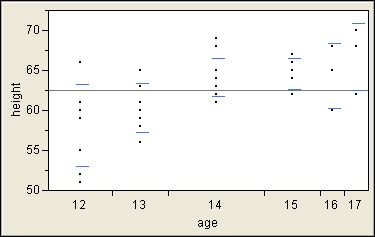2. JMP shows the location of the sample average with a diamond.

If you have a question about the location of each group, you can use the Means command. Once again, you get a new graphic in addition to a numerical report. The means diamonds present the sample averages as point estimates (center lines) and as confidence intervals (top and bottom points of the diamonds). The default confidence level is 95%. At any time, you can change this level with the Set Alpha Level command, and all of the reports are updated. In addition, the width of the diamond is proportional to the size of the group. This graphic is distinct from the previous standard deviation lines, and it is richer and gives a better answer about the location of each group.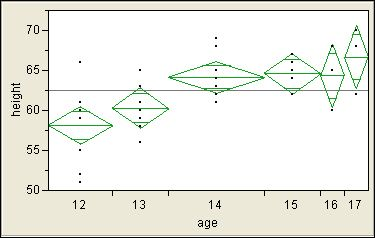3. JMP compares sample averages with circles.

If you want to compare the locations of groups, you can use the means diamonds again. They include overlap lines near the top and bottom. If the interval between these lines for one group does not overlap the interval between these lines for another group, then the group means are significantly different. These comparisons use the same significance level as the confidence interval, which is alpha = 0.05 by default.

The Munger's blog post mentioned a "rule of thumb," suggesting: "the confidence intervals can overlap by as much as 25% of their total length and still show a significant difference between the means for each group." First, note that the purpose of a confidence interval as such is to show the uncertainty in the group mean. The overlap lines, on the other hand, are specifically for such comparisons.

Second, we must also know that the error bars represent a confidence interval. Third, the assumptions for this rule are not entirely clear nor always met in practice. Fourth, the extent of overlap is difficult to assess visually. Another rule of thumb is suggested for the cases when the error bars represent standard error instead, and this rule suffers from similar weaknesses. If we use software to make a plot, why not also use it to make a better graphic and produce the proper hypothesis test and p-value, which is a better way to determine significance?

When you have more than two groups, though, the overlap lines provide no protection against the inflation of the experiment-wise alpha that is inherent in multiple comparisons. JMP provides a second way of comparing group averages that accounts for the number of comparisons to be made. Use one of the commands in the Compare Means group, such as the All Pairs, Tukey HSD command. This command produces a new graphic for each group. Like the means diamonds, the comparison circles inform you about the location (center) and spread (radius) of the sample statistics, but they are further adjusted to account for the number of comparisons. To compare group means, select a group by clicking on its circle, and JMP responds graphically with the answer; it is not enough to ascertain whether circles overlap.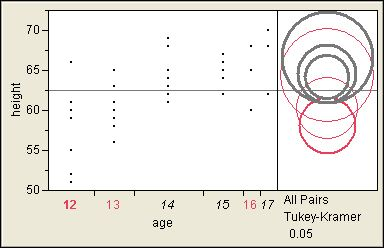I selected the lowest circle to ask whether this group (age=12) is different from any other groups in this example. The circles for three groups (age=14,15,17) change to gray to indicate that they are significantly different.

You can also use the connecting letters to determine which group averages are the same or different. Groups that do not share a letter are significantly different. Again you see that (age=12, group B) is different from (age=14,15,17, group A).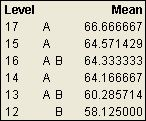So, what good are error bars? They are good when they clearly convey useful information in an unambiguous way. They might be over-taxed, though, and used for purposes for which better graphics have been invented. JMP considers the information that you want and uses the best graphic available to convey it.

I found another relevant example of error bars in JMP. When you use Fit Model to analyze a linear model with standard least squares regression, you automatically get a leverage plot for each term in the model if the Emphasis is Effect Leverage. (If you use another emphasis, you can still obtain the leverage plot by clicking the red triangle at the top of the window next to Response and selecting Row Diagnostics > Plot Effect Leverage. Now open the Effect Details and the reports for individual effects.) A categorical effect will include a Least Squares Means Table beneath the leverage plot. This report includes the LS mean, standard error, and mean for each level of this effect. You can right-click in the report and select Columns > Lower 95% and Columns > Upper 95% to get the limits for the 95% confidence interval of the LS means estimate. The result will look something like this: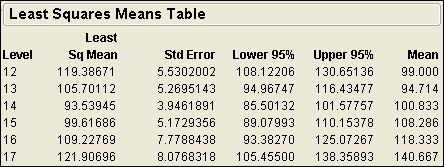(Note: this result uses Big Class data set and the model is weight = age + sex + height. This picture is for the age effect.)

Now click the red triangle at the top of the leverage plot and select LS Means Plot. The plot shows a graphic of the LS means along with error bars for the 95% confidence interval for comparison. The plot looks something like this: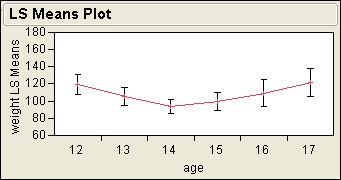Let me know if you find any other examples of error bars in JMP!

Error bars are optional in some JMP platforms. For example, you might want to show error bars in a bar chart. This example shows you how to add them.

1. Select Help > Sample Data. Open the Examples for Teaching section and select Big Class.

2. Select Graph > Chart. Select height > Statistics > Mean. Select age > Categories, X, Levels. Select OK.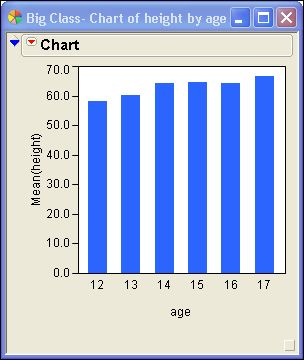3. Click the red triangle next to Chart and select Add Error Bars to Mean.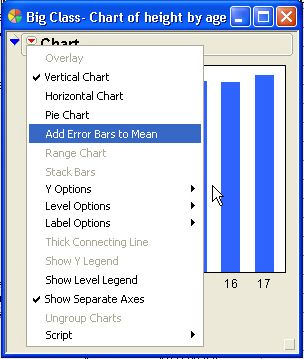4. You have choices about the quantity to use and its multiple. Use one standard error of the mean to show the uncertainty in its estimation.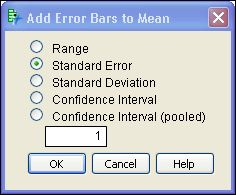5. Select OK.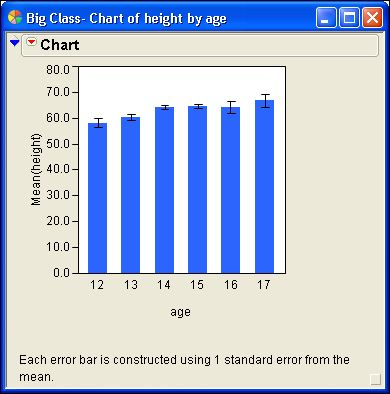That's all there is to it.

Article Labels

There are no labels assigned to this post.

Article Tags
Visitor

Mark Bailey wrote:

The long answer is that there are two kinds of populations involved in the analysis of your mixed effects model. The first kind is a data population, where we describe the spread of data values (e.g., response, predictor) using a standard deviation. The second kind is a statistic population, where we describe the spread of statistics (e.g., parameters, LS MEANS) using a standard error. The standard error is, in fact, the standard deviation of the sampling distribution of the estimated statistic.

The standard deviation of the data does not directly indicate the uncertainty of the estimated statistic, although it is related. The more the data vary (larger standard deviation), the more the sample statistic varies (larger standard error). The exact relation, however, depends on the model, its parameterization, and the method used to estimate the statistic. So the best indicator of the uncertainty for the statistic is its associated standard error.

Visitor

Elizabeth Salas wrote:

When using analyze-fit model to run a mixed-model 3-way ANOVA, all tables in the output shows Std Error. Is there any way of changing this to standard deviation?

Any help would be greatly appreciated!

Thanks

Visitor

Anger Management wrote:

Helpful information. Thank you very much.

Visitor

Mark Bailey wrote:

Visitor

Mark Bailey wrote:

Visitor

Florian wrote:

Does anyone know how I can add error bars on a bar graph in JMP?

Visitor

Mark Bailey wrote:

There is information about how the overlap marks in the means diamonds are computed. Refer to the JMP Statistics & Graphics Guide in the Chapter 7, which is all about the Oneway platform. (The reference manuals are available as PDF documents through the Help > Books menu.) See the section Means Diamonds and x-Axis Proportional in this chapter for more details.

Your transitivity example is not necessarily true, and I have seen exceptions to it. The letter groupings are meant to ease the interpretation of significant differences among the means for different levels. The letter designations work on the same principles as the underlying multiple comparison method upon which they are based. Simply put, if two groups share the same letter, then they are not signficantly different at the designated level. Otherwise, if two groups are assigned different letters, then they are signficantly different at the designated level. Some levels may be assigned to more than one letter.

Visitor

Walter R. Paczkowski wrote:

This was a good discussion of the error bars and the tools in JMP for making comparisons. I have two questions that are more for information or education. First, are there any references on the construction of the overlap regions of the diamonds? Second, the letter assignments can be confusing to interpret, so are there any guidelines for interpretation? For instance, are they transitive so that if X does not differ from Y and Y does not differ from Z, then does X not differ from Z?

Visitor

Mark Bailey wrote:

Your comment is much appreciated. Help us to help you! You (and any other reader) are welcome to suggest topics that might not occur to us or to indicate that a given topic should be a priority for us to cover here.

Visitor

Mark Bailey wrote:

We appreciate your comments. This blog is active and varied. We try to make it interesting to a wide audience. Please let us know if there are any particular issues or problems that interest you.

Visitor

dave wrote:

I'm a long time jump user but just recently found this blog. I want to say that posts such as this one are most interesting.

Visitor

Mark Bailey wrote:

This is another case where the comparison involved paired observations. In this case, if the observations are highly correlated or the variation between blocks is large, then the confidence interval of the sample averages will be greater than confidence interval for the paired differences. This case happens frequently. Unfortunately, many investigators do not recognized the pairing and use the less powerful two-sample test of the mean.

Once again, JMP provides a better solution: Analyze > Matched Pairs, if you recognize the pairing.

Visitor

Mark Bailey wrote:

I agree that the 95% confidence interval will be wider than an interval of one standard error because the critical t value for alpha=0.05 will always be greater than 1. However, if you required less confidence, the multiplier might be less than one. For example, if you only required 60% when comparing the means from two populations with a sample of 3 from each, your multiplier is only 0.94. This case is not likely, however.

Visitor

mid-level biological researcher wrote:

Thank you so much for this post. I have such a hard time explaining the meaning of error bars to coworkers, who are convinced that there is some sort of absolute standard for them. I am hoping that directing them to this post will help.

Visitor

Don Gregory wrote:

Also, the blog post author mentions that with correlated samples, error bars are inappropriate. I can understand the requirement that two â samplesâ be independent for anova, but if â beforeâ vs â afterâ measurements are not suitable, then I think there is a whole bunch of folks using the anova platform (with compare means) incorrectly?

I think there are a lot of folks who are using it for just that purpose; so the question is, if thatâ s not right, whatâ s the â correctâ way to evaluate a significance of a â beforeâ and â afterâ set of measurements?

Visitor

Don Gregory wrote:

The original blog post says â Standard errors are typically smaller than confidence intervals.â Assuming these are all normal distributions (not stated, but I think assumed), isnâ t it true that CI are always greater than the std.err. of the mean. I was thinking that the std.err. of the mean was the Std.Dev. of the population of the means of many samples (which is estimated from the data); and that the 95% CI would therefore be a multiple (approx 2 sigma) of the std.err?

Visitor

Top 10 JMP Blog posts of 2011 - JMP Blog wrote:

[...] What Good Are Error Bars? (2008) [...]

Visitor

Aris wrote:

Why is it necessary to use the overlap marks of the means diamonds to separate two means? Isn't the confidence interval overlap sufficient to show that two means are no different? Where does the formula 2^0.5 * CI/2 to calculate the overlap marks come from? It is not explained in the JMP manual.Staff

Mark Bailey wrote:

This is a great question, because it surfaces some common confusion about what these different intervals represent and for what use they are intended.

The confidence interval is a statement about the true mean. It conveys a given level of confidence about the location of the true mean. (It is not a statement about the probability that the true mean is within the interval.) It recognizes the uncertainty in our estimates of the sample statistics. The interval assumes that the true population mean and variance are fixed, and that the estimated mean and variance vary across simple random samples. It is computed as the point estimate with symmetric margins on either side for the uncertainty. The margin is a multiple (e.g., 1.96) of the standard error of the statistic. (The standard error measures the variation in a statistic like the standard deviation measures the variation in data.) The standard error is pooled from all of the groups because our analysis model provides a single, common variance. The multiplier assures that the desired proportion of cases (e.g., 95%) is represented by the interval. The multiplier is a quantile from the Student t distribution and a function of the group size.

This interval can be used for inference. It is equivalent to the one-sample version of Student's t-test. In this case, you are comparing a sample mean to a hypothesized population mean. If the interval contains the hypothesized mean, then you would not reject the null hypothesis. If the interval does not contain the hypothesized mean, then you would reject the null hypothesis and decide that the population mean is different from the hypothesized mean. The mean diamond represents both the point (middle horizontal line) and the interval estimates (vertical ends of the diamond). The one-sample t-test is based on a statistic that is computed as the difference between the sample mean and the hypothesized mean divided by the standard error of the mean.

We would not want to infer about the mean of one group using the confidence interval of another group as described immediately above. We can instead use a two-sample t-test, the equivalent one-way analysis of variance (ANOVA), or one of the four methods based on comparison circles. The two-sample t-test is based on a statistic that is computed as the difference between the sample means divided by the standard error of the difference of the sample means. The standard error of the difference can also be used to compute a confidence interval for the difference. The mean diamond represents this confidence interval as the overlap marks. The standard error of the difference is the square root of the sum of the squared standard errors of the group means. JMP assumes that the standard error of the mean is the same for all of the groups in order to simplify the computation of the margin: the square root of two times the square of the standard error of the mean. This assumption is true only when the groups have the same size, so this visual assessment should only be used in such cases.

For further information, please see the JMP Basic Analysis and Graphing guide (JMP > Help > Books) as well as this article in a 1996 issue of the JMPer Cable newsletter.. The article by Ann Lehman titled â The Value of Cut Diamondsâ discusses why the adjustment is appropriate.

Visitor

Christie wrote:

Does the mean error bars show the standard error mean?Staff

Mark Bailey wrote:

Yes, the variation in the sample statistic (mean estimate) is represented by the standard error of the mean and the standard error should be used to draw the error bars for the mean marker. This use of error bars should be explained by the author whenever it is used, instead of leaving it to the reader to assume or guess what the error bar represents.

Visitor

Emily wrote:

I read this entire post and although I am now very interested in statistics, I am still not clear on how to interpret error bars. I am a freshman at James Madison Univeristy studying biology, and I am currently encountering a lot of stats. issues when interpreting my lab results. Is this correct- the error bar tells you how from the mean your data distribution falls?Staff

Mark Bailey wrote:

I understand your confusion. That is common and the reason that I wrote this post in the first place. The answer, unfortunately, is that it depends on the quantity that is depicted by the error bar. (That dependence is why it is important that authors state what the error bar represents in a given plot.) If the error bar depicts one standard deviation, then it tells you about the typical difference between an observation and the mean. If it depicts one standard error, then it tells you about the typical difference between an estimate and the true parameter. If it depicts a confidence interval, then it tells you a range of values for the true parameter, with a given level of confidence.

From your comment, it seems that you were told to use one standard deviation of your lab data to plot the error bar. In this case, the error bar tells you the typical distance between an observation and the mean.